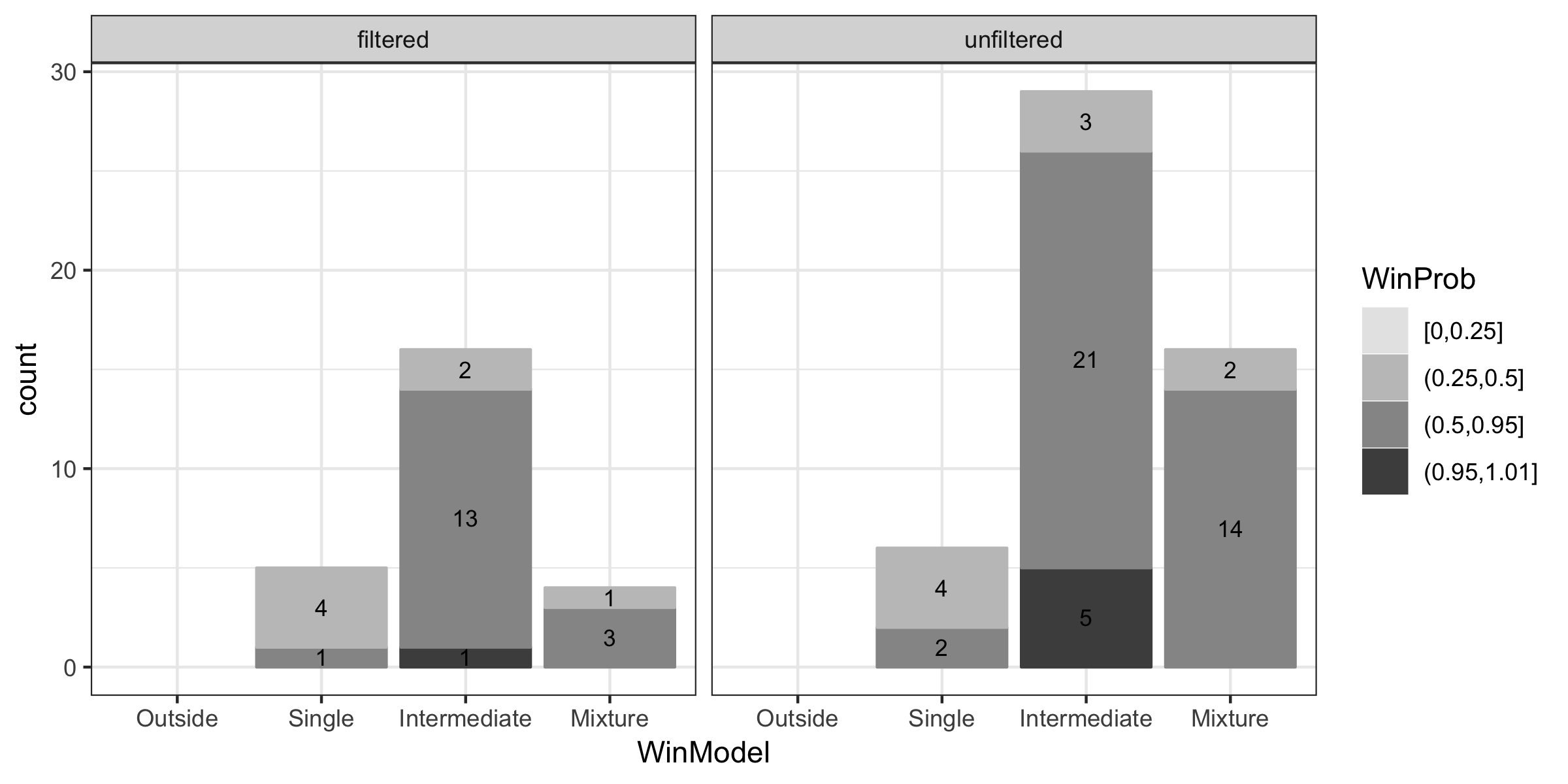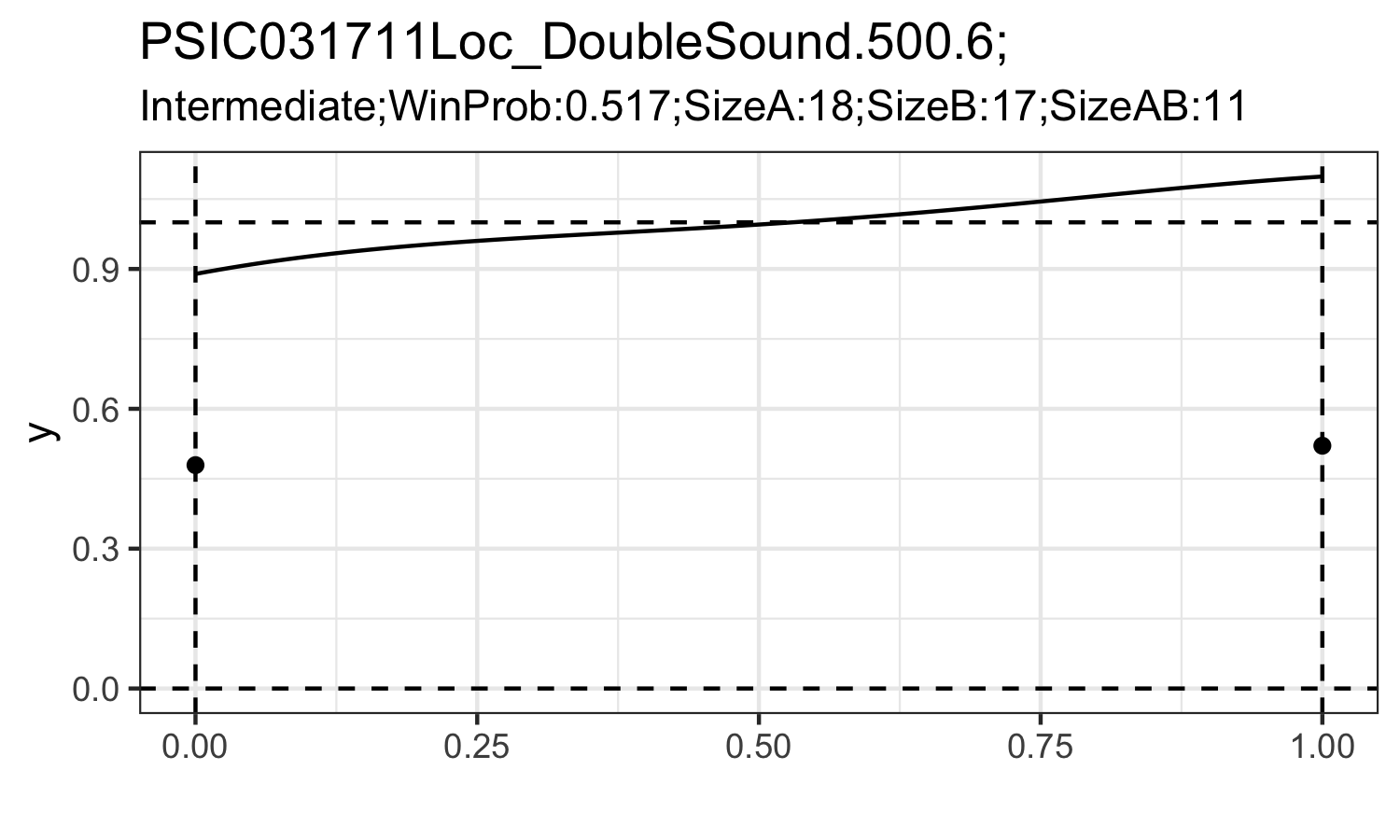## Overview

This package is designed to implement the PRML classifier and PRML filter proposed by Chen and Tokdar (2019).

• prml_filter() calculate Bayes factor of Poisson versus Poisson mixtures.
• prml_classifier() calculate posterior probabilities under each Poisson mixtures hypotheses.
• prml_classifier_f() calculate posterior probabilities under each Poisson mixtures hypotheses and density estimation of mixing density.
• prml_tests() output the result of PRML classifier prml_classifier() as well as PRML filter prml_filter()
• prml_tests_f() output the result of PRML classifier prml_classifier_f() as well as PRML filter prml_filter()

For real data anlaysis, we only need to use function prml_tests(), which take the $$\{Y^A,Y^B,Y^{AB}\}$$ as input. $$\{Y^A,Y^B,Y^{AB}\}$$ represent spike count data coming from the repeated trials under condition $$\{A,B,AB\}$$ (single-stimulus trial A, B and dual-stimuli trial AB). We provide a sample code on

1. how to extract the spike count triplets from the raw data;
1. how to use function prml_tests();
1. simple data visualization on the result.

If you want to obtain density estimation of the mixing density, replace prml_tests() with prml_tests_f() in the sample code. See Articles for details.

## Installation

Not yet available in cran. Install the development version for now.

install.packages("prml")

## Development version

devtools::install_github("yunranchen/prml")

## Result

A classification on the four possible hypotheses before PRML filtering and after PRML filtering process.A density estimation on the mixing density function. (Here only present the ‘Mixture’ and ‘Intermediate’ hypotheses.)